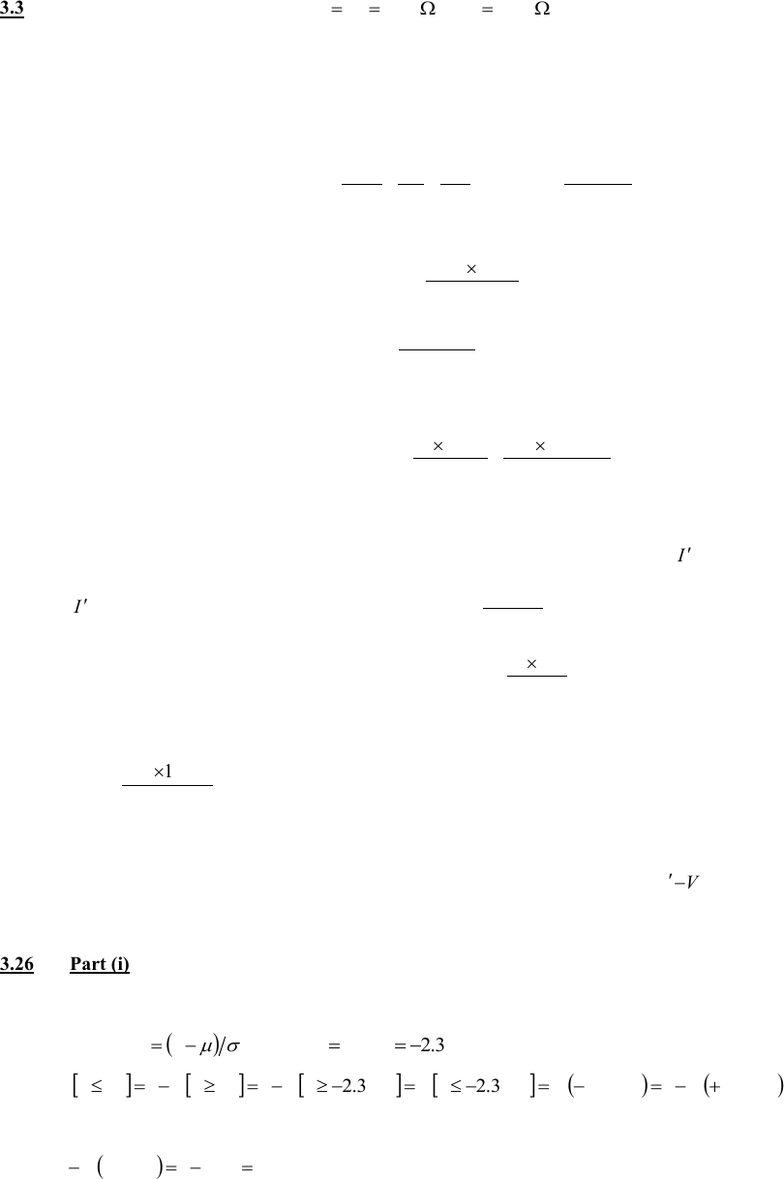Textbook Notes (280,000)
US (100,000)
LSU (20,000)
BE (50)
BE 2350 (20)
All (20)
Chapter

Solutions To Homework 2Exam

Department
Biological Engineering
Course Code
BE 2350
Professor
All

This preview shows half of the first page. to view the full 3 pages of the document.Solutions to Homework 2
3.3 Values given are: V = 20 Volts,
== 1000
21 RR
,
=9500
m
R
.
(i) Let the current flowing round the circuit with the voltmeter in position be I.
The resistance
AB
R
is composed of two resistances
2
R
and
m
R
in parallel. The value of
AB
R
can be calculated
from the formula for the effective resistance of two resistors in parallel:
mAB RRR
111
2
+=
i.e.
m
m
AB RR
RR
R+
=
2
2
Substituting in for the given numerical values of
2
R
and
m
R
:
=
+
=76.904
95001000
95001000
AB
R
According to Ohm’s law, I is given by:
Then the voltage across AB can be calculated from Ohm’s law as:
5.9
76.9041000
76.90420
1
=
+
=
+
==
AB
AB
ABAB RR
RV
RIV
volts
Thus the reading on the voltmeter is 9.5 volts.
(ii) Let the current flowing tround the circuit without the voltmeter in position be
I
.
I
can be calculated simply from Ohm’s law as:
21 RR
V
I+
=
The voltage across
2
V
can be calculated as
21
2
2RR
RV
RIV +
==
Substituting in numerical values:
0.10
10001000
100020 =
+
=
V
volts.
Thus, the voltage across AB when the voltmeter is not loading the circuit is 10.0 volts.
(iii) The measurement error due to the loading effect of the voltmeter is simply
AB
VV
, i.e. 0.5 volts.
3.26 Part (i)
Given data: = mean temperature = 75C ; = standard deviation = 2.15
Applying
( )
= xz
; For
3256.2,70 == zx
( ) ( )
3256.213256.23256.23256.2170170 +===== FFzPzPxPxP
Using error function (z-function or gaussian) table:
( )
1.099.013256.21 == F
Thus, the temperature is less than 70C for 1% of the time.
You're Reading a Preview

Unlock to view full version